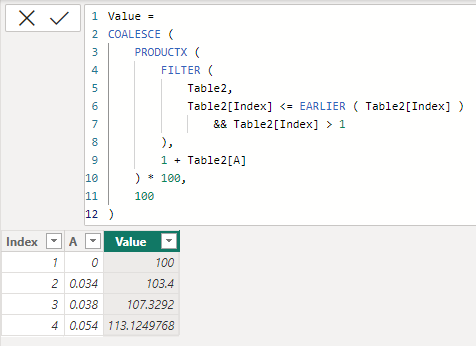cancel
Showing results for
Did you mean:Frequent Visitor

## How to get value from previous row in current calculated column

Hi,

I am trying to replicate the following calculation in DAX, where I want to get the value from the previous row in the current column (B).3 ACCEPTED SOLUTIONSSuper User

Hi @Pinecone
If B is a calculated column then

``````B =
SUMX (
FILTER ( 'Table', 'Table'[Index] <= EARLIER ( 'Table'[Index] ) ),
'Table'[A]
)``````Super User

Hi @Pinecone
Please refer to attached sample file with the proposed solution``````B =
COALESCE (
DIVIDE (
1,
PRODUCTX (
FILTER (
'Table',
'Table'[Index] <= EARLIER ( 'Table'[Index] )
&& 'Table'[Index] > 1
),
( 1 + 'Table'[A] ) ^ (1/16)
)
),
1
)``````

The numbers do not 100% match yours, but when I made the my own excel sample file, the numbers were 100% matching. Could it be a rounding error?Super User

@Pinecone``````Value =
COALESCE (
PRODUCTX (
FILTER (
Table2,
Table2[Index] <= EARLIER ( Table2[Index] )
&& Table2[Index] > 1
),
1 + Table2[A]
) * 100,
100
)``````
7 REPLIES 7Frequent Visitor

I have another similar issue.
Initial value in column C (C1) = 100
The following values in column C is:  C_i (C_i where i > 1) =  C_i-1 *(1+A_i)Super User

@Pinecone``````Value =
COALESCE (
PRODUCTX (
FILTER (
Table2,
Table2[Index] <= EARLIER ( Table2[Index] )
&& Table2[Index] > 1
),
1 + Table2[A]
) * 100,
100
)``````Super User

Hi @Pinecone
If B is a calculated column then

``````B =
SUMX (
FILTER ( 'Table', 'Table'[Index] <= EARLIER ( 'Table'[Index] ) ),
'Table'[A]
)``````Frequent Visitor

That did work fine. What if I want to expand the complexity by the following, knowing that B1 = 1,Super User

Hi @Pinecone
Please refer to attached sample file with the proposed solution``````B =
COALESCE (
DIVIDE (
1,
PRODUCTX (
FILTER (
'Table',
'Table'[Index] <= EARLIER ( 'Table'[Index] )
&& 'Table'[Index] > 1
),
( 1 + 'Table'[A] ) ^ (1/16)
)
),
1
)``````

The numbers do not 100% match yours, but when I made the my own excel sample file, the numbers were 100% matching. Could it be a rounding error?Frequent Visitor

Amazing, and thank you so much for solving my issue! ❤️Super User

Ya that is pretty complex. I'll have a look at it tomorrow.